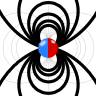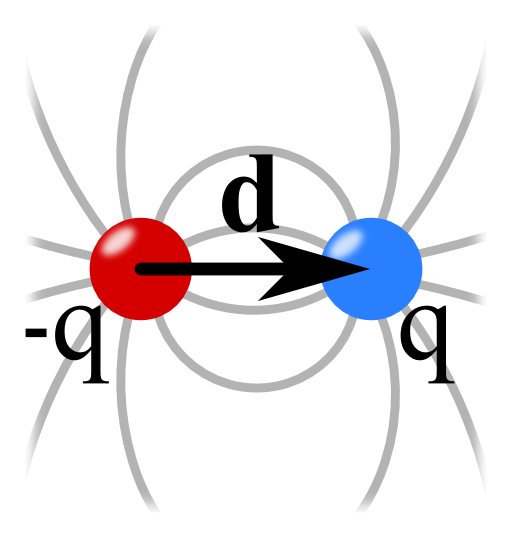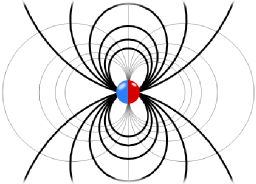## The Electric Field of a DipoleThe electric field of a dipole can be seen as the result of two charges approaching each other. Learn in this problem how to use the Taylor expansion of $$1/\left|\mathbf{r}-\mathbf{r}^{\prime}\right|$$ to calculate this field. Find out how this series expansion yields multipole moments, a very powerful description of the electric field.## Problem Statement

Find the electric field of an electric dipole using the following steps:

• Find the electrostatic potential of two point charges $$q_{1/2}=\pm q$$ at $$\mathbf{r}_{1/2}=\pm\frac{1}{2}\mathbf{d}$$.
• Take the limit $$\left|\mathbf{d}\right|\rightarrow0$$ of the potential holding $$\mathbf{p}:=q\mathbf{d}$$ constant.
• Calculate the electric field from the electrostatic potential.
• additional: Verify that the charge distribution $$\rho\left(\mathbf{r}\right)=-\mathbf{p}\cdot\nabla\delta\left(\mathbf{r}\right)$$ yields the correct dipole potential.

## Background: The Dipole as Limiting Process

As we have found in The Electric Field of two Point Charges, the electric field of two opposite charges is fundamentally different from that of a single charge decreasing with $$1/r^{3}$$ at large distances, not only with $$1/r^{2}$$. This is not just a coincidence but stems from the so-called multipole expansion as we have outlined earlier.

For now, let us try to find the dipole term by the limiting process of the given problem!

## Hints

The electrostatic potential of a point charge is given by (see again The Electric Field of a Point Charge)$\phi\left(\mathbf{r}\right) = \frac{1}{4\pi\varepsilon_{0}}\frac{q}{\left|\mathbf{r}-\mathbf{r}_{q}\right|}\ .$How can you calculate the field for several point charges?

Look at the arising potential for two charges. If you just insert $$\mathbf{d}=0$$, $$\phi\left(\mathbf{r}\right)$$ will simply vanish. Try to consider $$\phi\left(\mathbf{r}\right)$$ in terms of a series expansion in $$\mathbf{d}$$ to find its dependence of this parameter!

What is the relation between electrostatic field $$\mathbf{E}\left(\mathbf{r}\right)$$ and the electrostatic potential $$\phi\left(\mathbf{r}\right)$$?

For the additional part of of the problem, you will have to derive the $$\delta$$-distribution. Generally, acting on a test function $$\varphi$$, the derivative of a distribution is given by partia integration:$\int_{\mathbb{R}}\delta^{\prime}\left(x\right)\varphi\left(x\right)dx = -\int_{\mathbb{R}}\delta\left(x\right)\varphi^{\prime}\left(x\right)dx=-\varphi^{\prime}\left(0\right)\ .$

## Show Solution

The main steps to find the solution to this problem are first to figure out the electrostatic potential $$\phi\left(\mathbf{r}\right)$$ for a finite separation of the two charges. Then we have to use a Taylor expansion of the potential for small separations which will be outlined in detail. This will directly lead us to the potential of a dipole and its electric field.

The electrostatic potential for the two charges is given by a superposition of the potentials of each charge alone, $\begin{eqnarray*}\phi\left(\mathbf{r}\right)&=&\frac{1}{4\pi\varepsilon_{0}}\frac{q_{1}}{\left|\mathbf{r}-\mathbf{r}_{1}\right|}+\frac{1}{4\pi\varepsilon_{0}}\frac{q_{2}}{\left|\mathbf{r}-\mathbf{r}_{2}\right|}\\ & = &\frac{q}{4\pi\varepsilon_{0}}\left\{\frac{1}{\left|\mathbf{r}-\frac{1}{2}\mathbf{d}\right|}-\frac{1}{\left|\mathbf{r}+\frac{1}{2}\mathbf{d}\right|}\right\} \ .\end{eqnarray*}$

We now have to expand this potential in a Taylor series around $$\mathbf{d}=0$$. But let's take the chance to look at the Taylor expansion once again.

## The Taylor expansion of $$1/\left|\mathbf{r}-\mathbf{r}^{\prime}\right|$$

Remember that under certain assumptions of convergence, the Taylor expansion for a scalar function of one variable is given by $\begin{eqnarray*} f\left(x\right) & = & f\left(x_{0}\right)+f^{\prime}\left(x_{0}\right)\left(x-x_{0}\right)+\frac{1}{2}f^{\prime\prime}\left(x_{0}\right)\left(x-x_{0}\right)^{2}+\dots\\& = & \sum_{n=0}^{\infty}\frac{1}{n!}\left.\frac{d^{n}f\left(x\right)}{dx^{n}}\right|_{x_{0}}\cdot\left(x-x_{0}\right)^{n}\ ,\end{eqnarray*}$ or, in another familiar form as $\begin{eqnarray*}f\left(x-x_{0}\right) & = & \sum_{n=0}^{\infty}\frac{1}{n!}\left.\frac{d^{n}f\left(x-x_{0}\right)}{dx^{n}}\right|_{x=0}x^{n}\end{eqnarray*}$ using a little shift in the coordinate. For a function depending on several variables, it is better to use a notation with indices. For example with $$x_{1}=x$$, $$x_{2}=y$$ and $$x_{3}=z$$ we have (around $$\mathbf{r}^{\prime}$$) $\begin{eqnarray*}f\left(\mathbf{r}-\mathbf{r}^{\prime}\right) & = & f\left(\mathbf{r}\right)+\left.\frac{\partial f\left(\mathbf{r}-\mathbf{r}^{\prime}\right)}{\partial x_{1}}\right|_{\mathbf{r}=0}x_{1}+\\& & \left.\frac{\partial f\left(\mathbf{r}-\mathbf{r}^{\prime}\right)}{\partial x_{2}}\right|_{\mathbf{r}=0}x_{2}+\left.\frac{\partial f\left(\mathbf{r}-\mathbf{r}^{\prime}\right)}{\partial x_{3}}\right|_{\mathbf{r}=0}x_{3}\\& & +\mathcal{O}\left(\mathbf{r}^{2}\right)\end{eqnarray*}$using the second form of the Taylor expansion.

Here, however, we need an expansion of the potential around $$\mathbf{d}=0$$. So we have to treat $$\mathbf{d}$$ as the variable! To do so, we need an expansion of the function $$1/\left|\mathbf{r}-\mathbf{r}^{\prime}\right|$$ around $$\mathbf{r}^{\prime}=0$$ since the terms basically have this form with $$\mathbf{r}^{\prime}=\pm\frac{1}{2}\mathbf{d}$$. $\begin{eqnarray*}\frac{1}{\left|\mathbf{r}-\mathbf{r}^{\prime}\right|} & = & \frac{1}{\sqrt{\sum_{n=1}^{3}\left(x_{n}-x_{n}^{\prime}\right)^{2}}}\\& = & \left(\sum_{n=1}^{3}\left(x_{n}\right)^{2}\right)^{-1/2}+\sum_{k=1}^{3}\frac{\partial}{\partial x_{k}^{\prime}}\left. \left(\sum_{n=1}^{3}\left(x_{n}-x_{n}^{\prime}\right)^{2}\right)^{-1/2}\right|_{x_{k}^{\prime}=0}x_{k}^{\prime}+ \mathcal{O}\left(\mathbf{r^{\prime}}^{2}\right)\\& = & \frac{1}{r}+\sum_{k=1}^{3}\left.\left(-\right)2\left(x_{k}-x_{k}^{\prime}\right)\cdot\left(-\right)\frac{1}{2}\left(\sum_{n=1}^{3}\left(x_{n}-x_{n}^{\prime}\right)^{2}\right)^{-3/2} \right|_{x_{k}^{\prime}=0}x_{k}^{\prime}+\mathcal{O}\left(\mathbf{r^{\prime}}^{2}\right)\\& = & \frac{1}{r}+\frac{1}{r^{3}}\sum_{k=1}^{3}x_{k}x_{k}^{\prime}+\mathcal{O}\left(\mathbf{r^{\prime}}^{2}\right)=\frac{1}{r}+\frac{\mathbf{r}\cdot\mathbf{r}^{\prime}}{r^{3}}+\mathcal{O}\left(\mathbf{r^{\prime}}^{2}\right)\ .\end{eqnarray*}$Here we have used the chain rule $$\frac{d}{dx}\left(f\circ g\right)\left(x\right)=f^{\prime}\left(g\left(x\right)\right)g^{\prime}\left(x\right)$$. Note that it is also possible to derive this result using $$\frac{\partial}{\partial x_{k}^{\prime}}1/\left|\mathbf{r}-\mathbf{r}^{\prime}\right|=-\frac{\partial}{\partial x_{k}}1/\left|\mathbf{r}-\mathbf{r}^{\prime}\right|$$ as you may come across in the literature.

## Calculating the Electrostatic Potential and the Electric Field

We can apply our found expansion of the function $$1/\left|\mathbf{r}-\mathbf{r}^{\prime}\right|$$ using $$\mathbf{r}^{\prime}\rightarrow\pm\frac{1}{2}\mathbf{d}$$ to get an expression of the dipole potential for small separations: $\begin{eqnarray*}\frac{4\pi\varepsilon_{0}}{q}\phi\left(\mathbf{r}\right) & = & \frac{1}{\left|\mathbf{r}-\frac{1}{2}\mathbf{d}\right|}-\frac{1}{\left|\mathbf{r}+\frac{1}{2}\mathbf{d}\right|}\\& = & \frac{1}{r}+\frac{1}{2}\frac{\mathbf{d}\cdot\mathbf{r}}{r^{3}}-\frac{1}{r}+\frac{1}{2}\frac{\mathbf{d}\cdot\mathbf{r}}{r^{3}}+\mathcal{O}\left(\mathbf{d}^{2}\right)\\& = & \frac{\mathbf{d}\cdot\mathbf{r}}{r^{3}}+\mathcal{O}\left(\mathbf{d}^{2}\right)\ .\end{eqnarray*}$Now, we can perform the limit $$\mathbf{d}\rightarrow0$$ holding $$\mathbf{p}=q\mathbf{d}$$ constant to find the potential of a dipole as $\begin{eqnarray*}\phi\left(\mathbf{r}\right) & = & \frac{1}{4\pi\varepsilon_{0}}\frac{\mathbf{p}\cdot\mathbf{r}}{r^{3}}\ .\end{eqnarray*}$ Ok, we have made some nice progress up to now. We found the dipole potential from the somehow technical but extremely useful Taylor expansion of $$1/\left|\mathbf{r}-\mathbf{r}^{\prime}\right|$$. But we can still go a step further and calculate the electric field using $$\mathbf{E}\left(\mathbf{r}\right)=-\nabla\phi\left(\mathbf{r}\right)$$.

Now there is a long way to come to the desired result and a somewhat shorter one. The long way is to use that $\begin{eqnarray*}\frac{\mathbf{p}\cdot\mathbf{r}}{r^{3}} & = & -\mathbf{p}\cdot\nabla\frac{1}{r}\end{eqnarray*}\ ,$treat this product as vector product and use certain identities from vector calculus. I don't particularly like this. On the other hand we can also view $$\phi\left(\mathbf{r}\right)$$ as a product of scalar functions, namely $$\mathbf{p}\cdot\mathbf{r}$$ and $$r^{-3}$$. Then we know that $$\nabla\left(\mathbf{p}\cdot\mathbf{r}\, r^{-3}\right)=\mathbf{p}\cdot\mathbf{r}\nabla r^{-3}+r^{-3}\nabla\mathbf{p}\cdot\mathbf{r}$$. For the second term we see that $$\nabla\mathbf{p}\cdot\mathbf{r}=\mathbf{p}$$, since $$\mathbf{p}$$ is a constant vector. The other term is a little bit harder. In cartesian coordinates we calculate $\begin{eqnarray*} \nabla\frac{1}{r^{3}} & = & \underbrace{\sum_{i}\mathbf{e}_{i}\frac{\partial}{\partial x^{i}}}_{=\nabla}\underbrace{\left(\sum_{n}x_{n}^{2} \right)^{-3/2}}_{=r^{-3}}\\ & = & \sum_{i}\mathbf{e}_{i}2\delta_{in}x_{n}\left(-\frac{3}{2}\right)\left(\sum_{n}x_{n}^{2}\right)^{-5/2}\\ & = & -3\frac{\mathbf{r}}{r^{5}} \end{eqnarray*}$applying the chain rule.Putting everything together finally yields $\begin{eqnarray*}\mathbf{E}\left(\mathbf{r}\right) & = & -\nabla\phi\left(\mathbf{r}\right)=\frac{1}{4\pi\varepsilon_{0}}\left[3\left(\mathbf{p}\cdot\mathbf{r}\right)\frac{\mathbf{r}}{r^{5}}-\frac{\mathbf{p}}{r^{3}}\right]\ .\end{eqnarray*}$Note that this field is sometimes called a "mathematical dipole" and you might guess the reason for it. In the Taylor expansion we were just taking the linear term in $$\mathbf{d}$$. We have neglected all higher order terms. So the field we just found is a certain kind of approximation for distances larger than the dipole separation. We always have to remember how we came to a certain solution - the electric field will definately be different close to the dipole, see again The Electric Field of two Point Charges.

## Verification of $$\rho\left(\mathbf{r}\right)=-\mathbf{p}\cdot\nabla\delta\left(\mathbf{r}\right)$$

As given in the hints, we have to use the derivation of the $$\delta$$ distribution here. In one dimension it is given by$\int_{\mathbb{R}}\delta^{\prime}\left(x\right)\varphi\left(x\right)dx = -\int_{\mathbb{R}}\delta\left(x\right)\varphi^{\prime}\left(x\right)dx = -\varphi^{\prime}\left(0\right)\ ,$but we may apply this rule directly in three dimension since the result is the same. Ok, taking the integral formulation of Gauss's law$\begin{eqnarray*}\phi\left(\mathbf{r}\right)&=&\frac{1}{4\pi\varepsilon_{0}}\int\frac{\rho\left(\tilde{\mathbf{r}}\right)}{\left|\mathbf{r}-\tilde{\mathbf{r}}\right|}d\tilde{V} \\ &=& \frac{1}{4\pi\varepsilon_{0}}\int\frac{-\mathbf{p}\cdot\nabla_{\tilde{\mathbf{r}}}\delta\left(\tilde{\mathbf{r}}\right)}{\left|\mathbf{r}-\tilde{\mathbf{r}}\right|}d\tilde{V} \\ &=& \frac{1}{4\pi\varepsilon_{0}}\int\delta\left(\tilde{\mathbf{r}}\right)\mathbf{p}\cdot\nabla_{\tilde{\mathbf{r}}}\frac{1}{\left|\mathbf{r}-\tilde{\mathbf{r}}\right|}d\tilde{V} \\ &=& \frac{1}{4\pi\varepsilon_{0}}\int\delta\left(\tilde{\mathbf{r}}\right)\mathbf{p}\cdot\frac{\mathbf{r}-\tilde{\mathbf{r}}}{\left|\mathbf{r}-\tilde{\mathbf{r}}\right|^{3}}d\tilde{V} \\ &=& \frac{1}{4\pi\varepsilon_{0}}\frac{\mathbf{p}\cdot\mathbf{r}}{r^{3}}\ .\end{eqnarray*}$Note that $$\mathbf{p}$$ is just a constant vector such that $$\nabla_{\tilde{\mathbf{r}}}\mathbf{p}f\left(\tilde{\mathbf{r}}\right)=\mathbf{p}\cdot\nabla_{\tilde{\mathbf{r}}}f\left(\tilde{\mathbf{r}}\right)$$. Furthermore it is crucial here to take the derivative with respect to the coordinate of the charge distribution, $$\tilde{\mathbf{r}}$$! Otherwise we would get a sign change for the potential.

The verification of this charge distribution for a dipole is very useful. It provides a relation between the polarization of a dielectric object,$\mathbf{P}\left(\mathbf{r}\right) = \sum_{d}\mathbf{p}_{d}\delta\left(\mathbf{r}-\mathbf{r}_{d}\right)$and the charge density:$\begin{eqnarray*}\rho\left(\mathbf{r}\right)&=&-\sum_{d}\mathbf{p}_{d}\nabla\delta\left(\mathbf{r}-\mathbf{r}_{d}\right)\\&=&-\nabla\sum_{d}\mathbf{p}_{d}\delta\left(\mathbf{r}-\mathbf{r}_{d}\right)\\&=&-\nabla\mathbf{P}\left(\mathbf{r}\right)\ .\end{eqnarray*}$This relation will be extremely useful for our understanding of dielectric media in external electric fields.